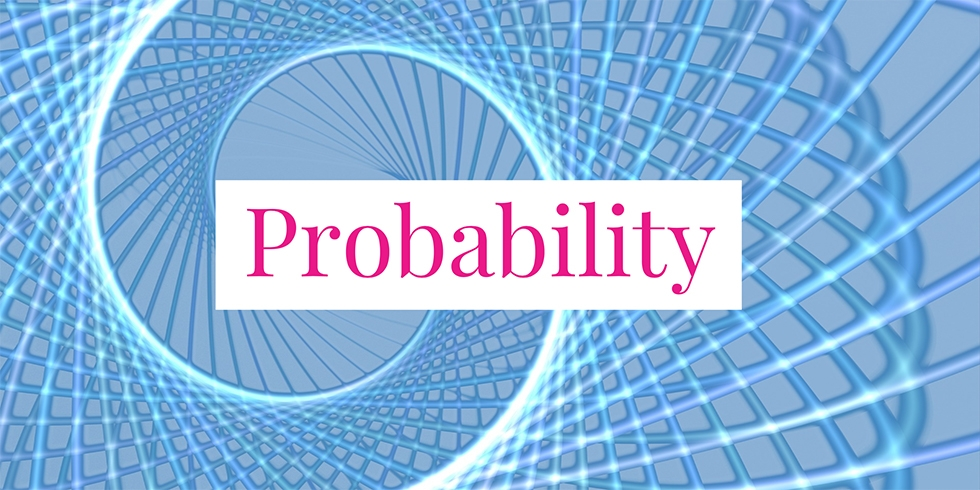# Pick Two Balls from a Box, What is the Probability Both are Red?## Problem 729

There are three blue balls and two red balls in a box.

When we randomly pick two balls out of the box without replacement, what is the probability that both of the balls are red?Add to solve later

## Solution.

Let $R_1$ be the event that the first ball is red and $R_2$ be the event that the second ball is red. Thus, the probability that both of the balls are red is given by $P(R_1 \cap R_2)$. By the product rule, we have
$P(R_1 \cap R_2) = P(R_1) \cdot P(R_2 \mid R_1).$

We have $P(R_1) = 2/5$ because we have a total of five balls of which two are red. Now, if the first ball is red, then the rest are three blue balls and one red ball. Hence the conditional probability $P(R_2 \mid R_1)$ is given by $P(R_2 \mid R_1) = 1/4$.

Combining these, we obtain
$P(R_1 \cap R_2) = \frac{2}{5} \cdot \frac{1}{4} = \frac{1}{10}.$ In conclusion, the probability that both of the balls are red is $1/10$.Add to solve later

### More from my site

#### You may also like...

This site uses Akismet to reduce spam. Learn how your comment data is processed.

###### More in Probability##### Conditional Probability Problems about Die Rolling

A fair six-sided die is rolled. (1) What is the conditional probability that the die lands on a prime number...

Close